# How to Sum Same Cell in Multiple Worksheets in Microsoft Excel

This post will guide you how to sum same cell in multiple worksheets with a formula in Excel. How do I sum same cell across multiple worksheets in Excel 2013/2016.

## Sum Same Cell in Multiple Worksheets

Assuming that you have three worksheets (1-Q, 2-Q, 3-Q) in your workbook, and you need to calculate the total value of certain cells (B1) in these worksheets. However, those cells are in several different worksheets. How can I sum up those cell values into Total worksheet. You can use the following formula:

`=SUM('1-Q'!B1, '2-Q'!B1, '3-Q'!B1)`

Or

`=SUM('1-Q:3-Q'!B1)`

Type this formula into a blank cell and press Enter key on your keyboard. You would get the total value of the same cell in those three worksheets.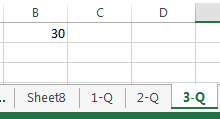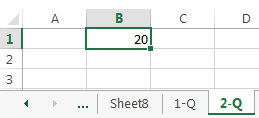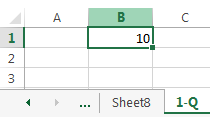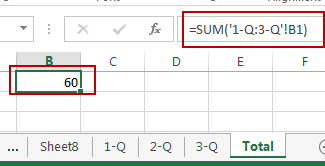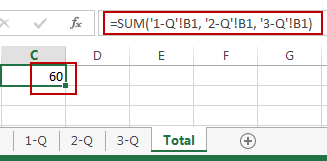Or you can also do the following steps to achieve the same result of calculating the total value of same cell across those 3 worksheets:

#1 go to Total worksheet, and select one blank cell that is the target cell that you want to place the calculating result.

#2 type =SUM( into the cell B1.

#3 select the tab of the first worksheet 1-Q.

#4 Hold the Shift key and then select the sheet tab of the last sheet 3-Q to sum().

Note: the above two steps will select the three target worksheets. And 1-Q is the first worksheet and 3-Q is the last worksheet. And other worksheets between these two will also be selected.

#5 select the cell B1 to sum. And then type )

#6 these actions will create the following formula, which can be used to sum up the same cell B1 from all three worksheets.

### Related Functions

• Excel SUM function
The Excel SUM function will adds all numbers in a range of cells and returns the sum of these values. You can add individual values, cell references or ranges in excel.The syntax of the SUM function is as below:= SUM(number1,[number2],…)…